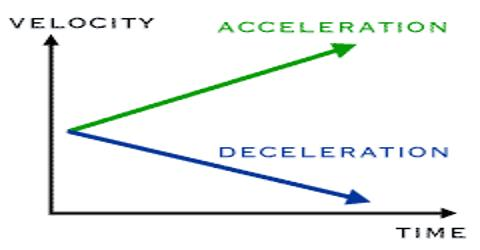# Velocity: Definition and Measurement

Velocity

Speed in a particular direction is called Velocity. The units for velocity can be implied from the definition to be meters/second or in general any distance unit over any time unit.

Velocity is a measure of the displacement of an object divided by the time it took to travel that distance. Suppose a man travels 15 meters in 10 seconds towards north. What will be her velocity?

Velocity = (displace/time) = (distance in a particular direction/time)

= 15 meters (towards-north) /10 seconds = 15 meters (m/s) (towards-north)

= 1.5 ms-1 (towards-north)

Direction of velocity should be mentioned with the magnitude of velocity. Because velocity has both magnitude and direction. But the speed has only magnitude.

The example of speed in the round-race of athletic competition where the distance travelled per second is the speed. It has no particular direction but has only magnitude. The distance travelled per second in a particular direction in the 100 meter sprint is the example of velocity.